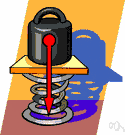# mass unit

Also found in: Thesaurus.
Related to mass unit: atomic mass unit, Mass formula
ThesaurusAntonymsRelated WordsSynonymsLegend:
 Noun 1mass unit - a unit of measurement for mass  unit, unit of measurement - any division of quantity accepted as a standard of measurement or exchange; "the dollar is the United States unit of currency"; "a unit of wheat is a bushel"; "change per unit volume"atomic mass unit - unit of mass for expressing masses of atoms or moleculesmass number, nucleon number - the sum of the number of neutrons and protons in an atomic nucleusavoirdupois unit - any of the units of the avoirdupois system of weightsmetric weight unit, weight unit - a decimal unit of weight based on the gramslug - a unit of mass equal to the mass that accelerates at 1 foot/sec/sec when acted upon by a force of 1 pound; approximately 14.5939 kilograms
Based on WordNet 3.0, Farlex clipart collection. © 2003-2012 Princeton University, Farlex Inc.
References in periodicals archive ?
"The change brings our best scientific understanding of the natural world directly into our daily lives, and gives us access to a definition of the mass unit which is available to everyone with the will and the skill to perform the experiments," Canada's chief metrologist, Alan Steele, told NBC News MACH in an email in advance of the vote.
The borderline ton-kilometer is a mass unit for transportation of cargo between borders by means of the railway transport.
At a given flow rate, the mean energy dissipation rate per mass unit can be regarded as a measure of turbulence at any point in the mixer.
Taking unit volume of the rock mass unit for energy analysis, it can be seen from the law of conservation of energy
In order to describe the collision process of the free mass unit intuitively and clearly and eliminate the interference factors, this paper analyzes the free mass collision process under no-load condition.
the mass spec signal may yield a "ladder" of mass signals separated by one C-U mass unit difference.
The Sartorius CPA Semi-Micro Balance has 5 built-in application programs which include counting, weighing, animal weighing, mass unit conversion, and net-total formulation.
The amount of dye adsorbed per mass unit of adsorbent, [q.sub.e], was calculated with equation qe = [([C.sub.i] - [C.sub.e])/[m.sub.a]] V, where, [C.sub.i] is the initial concentration of the dye in solution, [C.sub.e] is the concentration of the dye not adsorbed in solution in equilibrium, V is the solution volume, and [m.sub.a] is the mass of adsorbent used in the experiment.
where: [m.sub.0] is relative mass of the element at building without reserve (initial mass of the element without reserve); [k.sub.c,0] is the mass unit cost of the element at building; [m.sub.s,i] is the relative mass at i-type repair; [k.sub.c,s,i] is the mass unit cost at i-type repair.
With reference to the Planck mass [M.sub.p] [congruent to] [square root of [??]c/G] and the elementary charge e, a new mass unit [M.sub.c] [congruent to] [MATHEMATICAL EXPRESSION NOT REPRODUCIBLE IN ASCII] can be constructed.

Site: Follow: Share:
Open / Close